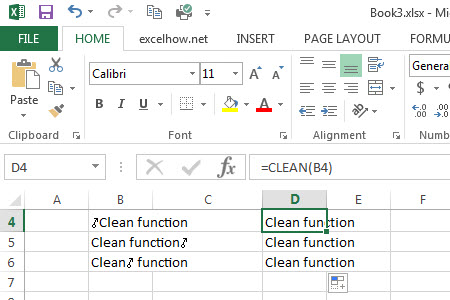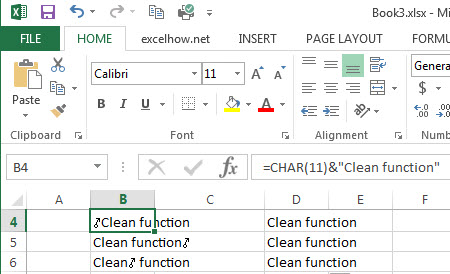# Excel Clean Function

This post will guide you how to use Excel CLEAN function with syntax and examples in Microsoft excel.

### Description

The Excel CLEAN function removes all non-printable characters from a text string.

The CLEAN function is a build-in function in Microsoft Excel and it is categorized as a Text Function.

The CLEAN function is available in Excel 2016, Excel 2013, Excel 2010, Excel 2007, Excel 2003, Excel XP, Excel 2000, Excel 2011 for Mac.

### Syntax

The syntax of the CLEAN function is as below:

= CLEAN  (text)

Where the CLEAN function argument is:
Text – this is a required argument. The text strings that you want to remove all nonprintable characters

### Example:

The below examples will show you how to use Excel Clean Text function to clean all non-printable characters, such as:

#1 To remove all vertical tab characters from text string in B4-B6 cells, just using CLEAN(B4), CLEAN(B5), CLEAN(B6) formulas.You will get the same results after removing non-printable characters.Related Posts

Excel DVARP Function

This post will guide you how to use Excel DVARP function with syntax and examples in Microsoft excel. Description The Excel DVARP Function will get the variance of a population based on the entire population of numbers in a column ...

Excel DVAR Function

This post will guide you how to use Excel DVAR function with syntax and examples in Microsoft excel. Description The Excel DVAR Function will get the variance of a population based on a sample of numbers in a column in ...

Excel DSUM Function

This post will guide you how to use Excel DSUM function with syntax and examples in Microsoft excel. Description The Excel DSUM Function will add the numbers in a column or database that meets a given criteria. And so you ...

Excel DSTDEVP Function

This post will guide you how to use Excel DSTDEVP function with syntax and examples in Microsoft excel. Description The Excel DSTDEVP returns the standard deviation of a population based on the entire population of numbers in a column or ...

Excel DSTDEV Function

This post will guide you how to use Excel DSTDEV function with syntax and examples in Microsoft excel. Description The Excel DSTDEV returns the standard deviation of a population based on a sample of numbers in a column or database ...

Excel DMIN Function

This post will guide you how to use Excel DMIN function with syntax and examples in Microsoft excel. Description The Excel DMIN returns the minimum value from a database or a column of a list that matches the specified conditions. ...

Excel DPRODUCT Function

This post will guide you how to use Excel DPRODUCT function with syntax and examples in Microsoft excel. Description The Excel DPRODUCT returns the product of values from a set of records that match criteria that you specify. The DPRODUCT ...

Excel XIRR Function

This post will guide you how to use Excel XIRR function with syntax and examples in Microsoft excel. Description The Excel XIRR function returns the internal rate of return for a series of cash flows that is not necessarily periodic. ...

Excel VDB Function

This post will guide you how to use Excel VDB function with syntax and examples in Microsoft excel. Description The Excel VDB function calculates the depreciation of an asset for a specified period based on the double declining balance method. ...

Excel SYD Function

This post will guide you how to use Excel SYD function with syntax and examples in Microsoft excel. Description The Excel SYD function calculates the sum-of-years’digits depreciation of an asset for a specified period. And it will return a numeric ...

Sidebar